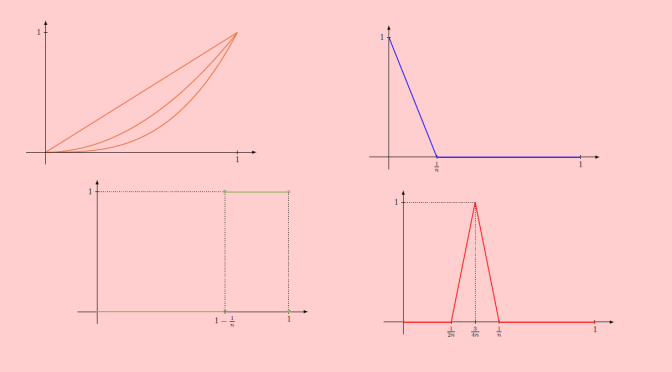# Counterexamples around Dini’s theorem

In this article we look at counterexamples around Dini’s theorem. Let’s recall:

Dini’s theorem: If $$K$$ is a compact topological space, and $$(f_n)_{n \in \mathbb N}$$ is a monotonically decreasing sequence (meaning $$f_{n+1}(x) \le f_n(x)$$ for all $$n \in \mathbb N$$ and $$x \in K$$) of continuous real-valued functions on $$K$$ which converges pointwise to a continuous function $$f$$, then the convergence is uniform.

We look at what happens to the conclusion if we drop some of the hypothesis.

### Cases if $$K$$ is not compact

We take $$K=(0,1)$$, which is not closed equipped with the common distance. The sequence $$f_n(x)=x^n$$ of continuous functions decreases pointwise to the always vanishing function. But the convergence is not uniform because for all $$n \in \mathbb N$$ $\sup\limits_{x \in (0,1)} x^n = 1$

The set $$K=\mathbb R$$ is closed but unbounded, hence also not compact. The sequence defined by $f_n(x)=\begin{cases} 0 & \text{for } x < n\\ \frac{x-n}{n} & \text{for } n \le x < 2n\\ 1 & \text{for } x \ge 2n \end{cases}$ is continuous and monotonically decreasing. It converges to $$0$$. However, the convergence is not uniform as for all $$n \in \mathbb N$$: $$\sup\{f_n(x) : x \in \mathbb R\} =1$$.

### Counterexample if $$f$$ is not continuous

We take $$K=[0,1]$$ which is compact and the sequence $f_n(x)=\begin{cases} 1 – 2nx & \text{for } 0 \le x < \frac{1}{2n}\\ 0 & \text{for } \frac{1}{2n} \le x \le 1 \end{cases}$ One can verify that $$f_n$$ is continuous for all $$n \in \mathbb N$$. The sequence $$(f_n)$$ is decreasing and converges pointwise to the discontinuous function equal to $$1$$ at $$0$$ and to $$0$$ elsewhere. However, again the convergence is not uniform as for all $$n \in \mathbb N$$: $$\sup\{f_n(x) : x \in [0,1]\} =1$$.

### Counterexample if $$(f_n)$$ is not monotonically increasing or decreasing

We keep $$K=[0,1]$$ and consider the sequence $f_n(x)=\begin{cases} 0 & \text{for } 0 \le x < \frac{1}{2n}\\ 4nx-2 & \text{for } \frac{1}{2n} \le x < \frac{3}{4n}\\ 4-4nx & \frac{3}{4n} \le x < \frac{1}{n}\\ 1 & \text{for } \frac{1}{n} \le x \le 1 \end{cases}$ One can verify that $$f_n$$ is continuous for all $$n \in \mathbb N$$. The sequence $$(f_n)$$ converges pointwise to $$0$$. However, again the convergence is not uniform as for all $$n \in \mathbb N$$: $$\sup\{f_n(x) : x \in [0,1]\} =1$$.

### Counterexample if the $$f_n$$ are not continuous

We consider again $$K=[0,1]$$ and the sequence $f_n(x)=\begin{cases} 0 & \text{for } 0 \le x < 1-{1\over n}\\ 1 & \text{for } 1-{1\over n} \le x < 1\\ 0 & \text{for } x=1 \end{cases}$ $$(f_n)$$ is a sequence of non continuous functions, converging pointwise to $$0$$. The convergence is not uniform as for all $$n \in \mathbb N$$: $$\sup\{f_n(x) : x \in [0,1]\} =1$$.